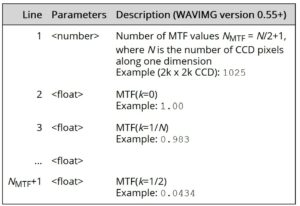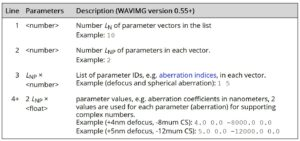# Dr. Probe

### HR(S)TEM image simulation package

###### CellMuncher

The program WAVIMG calculates high-resolution TEM images from electron wavefunctions.

###### Options
 Line Parameters Description (Version 0.55+) 01 File name of the input wave functionExample: `'wav\exit-wave.dat'` 02 , Number of columns and rows (samples) of the input wave function [pixels]Example: `192, 256` 03 , Physical row and column sampling rate of the input wave function [nm/pixel]Example: `0.003878, 0.003727` 04 Primary electron energy [keV]Example: `300.0` 05 Switch option for the type of output: 0 = TEM image 1 = wave function 2 = w.f. amplitude 3 = w.f. phase 4 = w.f. real part 5 = w.f. imaginary part 6 = TEM image map from a 2D parameter variation Example: `0` 06 File name of the output image or wave function dataExample: `'img\TEM-image.dat'` 07 , Number of columns and rows (samples) of the output image [pixels]Example: `64, 72` 08 , , , Image output data type, image vacuum mean intensity [counts], conversion rate [counts/e-], readout noise rms amplitude [counts] Image data types: 0 = 32-bit float, 1 = 32-bit integer, 2 = 16-bit integerExample (default simulation): `0, 1.0, 1.0, 0.0`Example (simulation with noise): `1, 3451.2, 5.1, 3.0` 09 Switch SF for extracting a specific image frameSF = 0: OFF, SF = 1: ONThe parameters defined in lines 10 – 12 are ignored when SF = 0.Example: `1` 10 Image sampling rate [nm/pixel]Applies only if SF = 1Example: `0.008973` 11 , Image frame offset in pixels of the input wave functionsApplies only if SF = 1e.g. `13.4, 16.2` 12 Image frame rotation with respect to the input wave horizontal axis [deg]Applies only if SF = 1Example: `0.0` 13 Switch option for selecting the model for the simulation of partial coherence in the image formation. Numbers from 1 to 6, see table of supported coherence models below. Example: `1` 14 , Partial temporal coherence switch and focus-spread (1/e-half width) [nm]Set the switch = 0 to deactivate and = 1 to activate the simulation of partial temporal coherenceExample: `1, 3.8` 15 , Partial spatial coherence switch and semi-convergence angle (1/e-half width) [mrad]Set the switch = 0 to deactivate and = 1 to activate the simulation of partial spatial coherenceExample: `1, 0.4` 16 , , Switch, k-space scaling, and file name for the simulation of a frequency modulated detector transfer function (MTF)Set the switch = 0 to deactivate and = 1 to activate the MTF simulation.The k-space scaling should be equal to the ratio of sampling rates of experiment and simulation (sexp/ssim).Example: `1, 1.0, 'C:\data\CCD-MTF-300kV.dat'` 17 , , , Switch and rms amplitudes [nm, nm, deg] of the effective image spread.Set the switch = 0 to deactivate or = 1 or = 2 to activate the simulation of an image spread envelope.Switch = 1 assumes an isotropic image spread defined by one rms amplitude.Switch = 2 assumes an anisotropic image spread defined by two perpendicular major rms amplitudes and an azimuth orientation angle. The orientation determines the direction of the first major vibration axis with respect to the horizontal image axis.Example: `1, 0.016` 18 Number Nabr of image aberrations set belowYou have to provide exactly this number of aberration definitions in the following lines. Example: `2` 18+ , , Aberration definition by an index number and two coefficient values given in [nm] (details)Nabr aberration definitions are expected following line 11. By default aberration coefficient values are all zero. You may set Nabr = 0 and leave out all aberration definitions. Note that depending on the number of aberration definitions Nabr, the following content is moved further lines down in the parameter file. 19+ Nabr Objective aperture radius [mrad]Example: `250.0` 20+ Nabr , Center of the objective aperture with respect to the zero beam [mrad, mrad]Example: `0.0, 0.0` 21+ Nabr Number of parameter loops Nlp defined below.At least Nlp loop definitions have to follow this line. Since each loop definition itself requires 5 lines, we restrict the description here to 1 loop definition. When more than one loop is defined, the first loop definition in the parameter file will be the innermost loop of a nested loop calculation.As the parameter file ends after the loop definitions, you may simply switch off all parameter loops by setting Nlp = 0.Example: `1` 22+ Nabr Loop parameter class Lc. 1 = coherent aberration 2 = incoherent aberration 3 = input wave function file Example: `1` 23+ Nabr Loop parameter index LpExample (defocus): `1` 24+ Nabr Loop variation form Lf 1 = linear ramp 2 = cosine oscillation 3 = list directed aberration parameters Example: `1` 25+ Nabr , , Loop range (Lr0, Lr1, Lrn) case Lf = 1: Lr0 = min., Lr1 = max., Lrn = number of samples case Lf = 2: Lr0 = amplitude, Lr1 = frequency, Lrn = number of samples case Lf = 3: ignored, parameter values are taken from file Example: `0.0, 10.0, 21` 26+ Nabr Loop string identifier Ls This string is used very flexible depending on the loop class and form: Lc = 3: The string Ls specifies the exact substring preceding the index in a loop over input wave function file names.For example set Ls = `'_sl'` to address the wave function files of a thickness series obtained with MSA, such as `'wave_sl001.dat'`, `'wave_sl002.dat'`, `'wave_sl003.dat'`, etc. Lf = 3: The string Ls specifies the exact file name describing a list directed parameter variation. Example: `'_sl'`

#### Parameter and Control Files

Microscope, imaging, and calculation control parameters of the program WAVIMG have to be provided by a parameter file. Parameter files are text files containing a list of values and strings in a fix sequence. The table below describes the structure of the parameter file and provides short descriptions. A parameter file must be provided when calling the `wavimg.exe` application.

##### List of coherence models for image simulations

 Switch Coherence model 1 Non-linear image calculation including partial temporal coherence (focus spread) by explicit averaging. Partial spatial coherence is treated by a quasi-coherent approach with a dampening envelope applied to the wave function. This model is good for HRTEM with Cs-corrected microscopes, where the partial spatial coherence can be largely ignored but the partial temporal coherence limits the resolution as a compromise for faster calculation. 2 Non-linear image calculation by explicit averaging of coherent sub-images with focal variation and angular variation. With sufficient numbe rof focal and angular samples, this is a fully accurate calculation of partial coherence including all cross-terms and non-linearities. It is valid for all kinds of TEMs but quite time consuming. 3 Linear image calculation considering partial coherence by linear envelopes applied to the linear interference terms. This approximation remains valid for weak-phase objects. Non-linear image components are ignored. 4 Fourier-space synthesis with partially coherent transmission cross-coefficient (TCC). This is a fully non-linear imaging model with dampening including a first-order derivative solution of the TCC. It can be applied to moderate coherence cases. The calculation is very time consuming and only implemented for reference. Mode 2 should be preferred over mode 4. 5 Non-linear image calculation including frozen-lattice variations with explicit averaging of focal and angular distributions for partial coherence. The averaging is over random samples of the distribution functions. 6 Non-linear image calculation in a quasi-coherent approximation of partial coherence. A dampened wave function in the image plane is calculated by multiplying linear dampening envelopes in Fourier space and then calculating the absolute square in real space. This approximation applies a too strong dampening to some of the non-linear interference terms. It is of limited use and valid only for very thin samples or microscopes where the resolution is not limited by partial coherence effects.
##### MTF File

Line 16 of the WAVIMG parameter file you specifies a detector MTF by a file name. The MTF file is a text list of values describing the frequency dependent amplitude modulation of the image contrast in a radially symmetric form. The MTF data is assumed to be given without the attenuation due to pixel binning.

The format of the MTF text file is described in the table below.The MTF may be different for each detector and can vary significantly with the electron energy. If possible the MTF should be measured with a knife edge or similar method. The measured MTF of a Gatan UltraScan 1000 with 2k x 2k pixels for 300 keV electrons is provided here as an example.

Download MTF of a Gatan UltraScan 1000P TEM CCD detector for 300 keV electrons.

##### Parameter Variation List

The list directed parameter loop form allows you to apply a completely flexible form of multiple parameter variation. Since version 0.55 of WAVIMG, this list can be used to vary the image aberrations in an arbitrary way.

An additional parameter file is used to input a list of parameter variation vectors. A parameter vector is applied with each step of the respective parameter loop in the provided sequence. Aberration vectors specified in the file are added to the parameter setting given by the main WAVIMG parameter file. Multiple list directed parameter variations are added up.

The format of the parameter variation list is described in the table below.